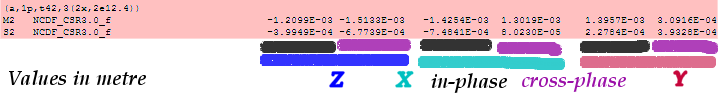Main page | FAQ's | References | Short loading course | OSO homepage

## Correction for the motion of the centre of mass of the solid Earth

When the solid Earth and oceans are considered to be a system without any external forces on it, then the position of its common centre of mass will remain fixed in space. Since the ocean tides cause water mass displacements, its centre of mass will move periodically and must be compensated by an opposite motion of the centre of mass of the solid Earth. Our stations are placed on the solid earth, thusthey are subject to this counter-motion.

Farrell (1972) was aware of this phenomenon as he laid out the basis for the loading Green's functions. The resolution was that the loading effects are reckoned in a reference frame that is attached to solid Earth. For example, a gravimeter that is measuring at the Earth surface will be insensitive to translations of the solid earth mass centre. On the other hand, space geodetic techniques such as Satellite Laser Ranging (SLR), see Watkins and Eanes (1997), and the Global Positioning System (GPS) are fixed in space and should in principle be able to detect this centre of mass motion of the solid Earth. Therefore the option has been added to include this motion into the ocean tide loading values.

Scherneck (2000) warns that during GPS orbits determination, it is possible that this large scale motion can be absorbed in the GPS satellite positions. In this case the correction for the centre of mass motion of the solid Earth motion has indirectly been applied.

Please make sure that you apply the centre of mass terms consistently. And don't be surprised when something you put in at the one end comes out at the other. (Applies to certain conducts like tunnels, wires, and satellite ranges, but not sausages like isterband.)

The centre of mass motions that are added when you check YES hereare computed from the same set of tide waves as the loading coefficients. So in principle each displacement coefficient is changed.

We offer the center of mass coefficient files for the different tide models. They are formatted as follows:

``
Usage: t=time, angle=atronomical tide argument
`DX(t) = Sum(n in 11 tides) Xin(n) * COS(angle(t,n)) + Xcr(n) * SIN(angle(t,n))DY(t) = Sum(n in 11 tides) Yin(n) * COS(angle(t,n)) + Ycr(n) * SIN(angle(t,n))DZ(t) = Sum(n in 11 tides) Zin(n) * COS(angle(t,n)) + Zcr(n) * SIN(angle(t,n))`

[DX,DY,DZ] is the offset of the terrestrial, solid-earth fixed frame reckoned from the joint mass centre (solid earth + tidal ocean), and are given in the geocentric cartesian system.

You can obtain CMC parameters in the following two variants
1. CMC with a correction for tidal mass conservation (that was the standard upto July 29, 2011
2. CMC_MIB without (`_MIB´ signifying the mass imbalance implied)
You can see the adjustment (uniform, co-oscillating slab with cos and sin components) in the
collection of mass imbalances. The format is explained as follows:

_____________________________________________________________________________________

Model      Tide                       COS         SIN
_____________________________________________________________________________________

SCHW       M2    <C_Mass>>> Corr.=  6.4374D-03 -3.4342D-03 m, data max.=  4.00D+00 m

CAVEAT EMPTOR:
I am currently in a discussion with a colleague, and we are in substantial disagreement as to the validity of these parameters. Don't worry, we keep the discussion in a real friendly spirit. That part of it is fun. The suspicion that you may get very bad numbers is of course a bring-down. Well, some might like to get just any number provided it is a number and not NaN or ****** - feel happy! My view of this whole stuff, however, can be expressed as follows:

I offer a bottle of champagne, a real good one, value at €50, or, if you refrain from such beverages, a mineral specimen (e.g. a meteorite, an amber, an opal) of equivalent value, if you can can prove that one model's CMC set reduces GPS (or any other satellite analysis') errors in e.g. tide parameters or station parameter variance or any kind of WRMS-of-fit more than another. The fat words constrain the wager.  Don't forget that the CMC translations may or may not have been applied in the orbit determination/adjustment.
You must show that space and ground segment data modelling is consistent with respect to the model from which the CMC are derived.
You get a double-value reward if you can compute better CMC coefficients than me